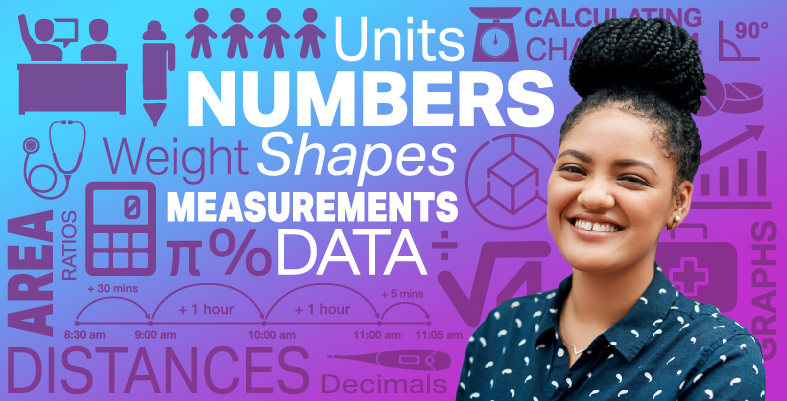Everyday maths for Health and Social Care and Education Support 2

Start this free course now. Just create an account and sign in. Enrol and complete the course for a free statement of participation or digital badge if available.

Free course

# 6.3 Finding the mean from a set of data

To find the mean of a simple set of data, all you need to do is find the total, or sum, of all the items together and then divide this total by how many items of data there are.

## Table 11 (repeated)

MondayTuesdayWednesdayThursdayFriday
74363

Look again at the number of missed appointments at one GP practice (shown above). If you want to calculate the mean average of missed appointments, you first find the sum or total of the weight missed appointments for the week:

• 7 + 4 + 3 + 6 + 3 = 23

Next, divide this total but the number of data items, in this case, 7:

• 23 ÷ 5 = 4.6

It is important to note that the mean may well be a decimal number even if the numbers you added together were whole numbers.

Another important thing to note here is that the two sums (the addition and then the division) are done as two separate sums. If you were to write:

• 7 + 4 + 3 + 6 + 3 ÷ 7

this would be incorrect (remember BIDMAS from Session 1?). Unless you are going to use brackets to show which sum needs to be done first (7 + 4 + 3 + 6 + 3) ÷ 7, it is accurate to write two separate calculations.

Have a go at calculating the mean for yourself by completing the activity below.

## Activity 10: Finding the mean

1. The table below shows the sale price of ten, 2 bedroom semi-detached houses in a town in Liverpool.

### Table 14

House 1House 2House 3House 4House 5House 6House 7House 8House 9House 10
£70,000£65,950£66,500£71,200£68,000£62,995£70,500£68,750£59,95067,900
• What is the mean house price in this area?

1. The table below shows the number of hours of childcare provided by a childminder each month.

### Table 15

JanuaryFebruaryMarchAprilMayJune
12010513298101134
• Calculate the mean amount of hours per month.

1. First find the total of the house prices:

• £ 70,000 + £ 65,950 + £ 66,500 + £ 71,200 + £ 68,000 + £ 62,995 + £ 70,500 + £ 68,750 + £ 59,950 + £ 67,900 = £ 671,745

Now divide this total by the number of houses (10):

• £671,745 ÷ 10 = £67,174.50

1. Find the total number of units used:

• 120 + 105 + 132 + 98 + 101 + 134 = 690 hours

Now divide this total by the number of months (6):

• 690 ÷ 6 = 115 hours

This method of finding the mean is fine if you have a relatively small set of data. What about if the set of data you have is much larger? If this was the case, the data would probably not be presented as a list of numbers, it’s much more likely to be presented in a frequency table.

In the next part of this section, you will learn how find the mean when data is presented in this way.

FSM_SSH_2